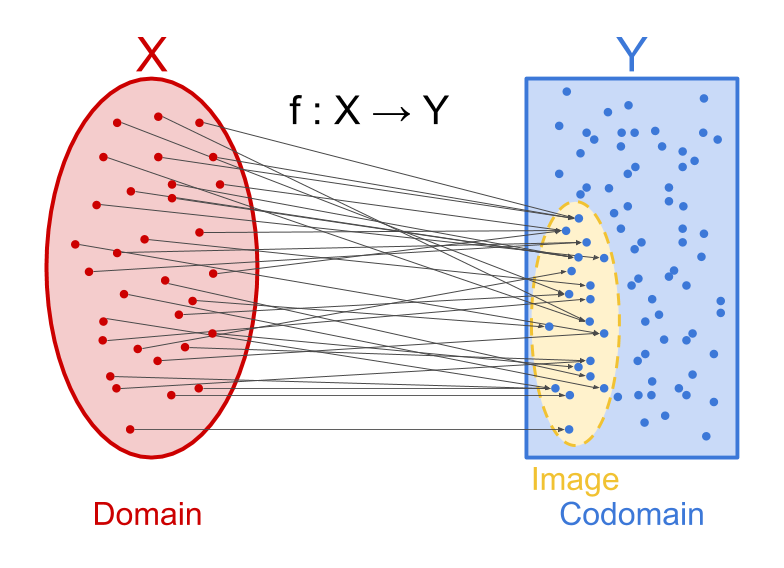# Codomain vs image

A function $$f : X \to Y$$ with domain $$X$$ and codomain $$Y$$ cannot necessarily produce all values in $$Y$$. For example, consider the function that takes a real number and squares it. We could say that this function has codomain $$\mathbb R$$, because it produces a real number, even though its image is the set of non-negative real numbers.If $$f$$ maps $$X$$ to a subset $$I$$ of $$Y$$, why not just say that its codomain is $$I$$ and do away with the distinction between codomain and image? There are at least two cases where the distinction is useful.

1. It is useful to distinguish codomain from image in cases where the type of thing that the function produces is easy to specify, but the specific values are hard to calculate. Consider, for instance, the Ackermann function. It definitely produces numbers (i.e. its codomain is $$\mathbb N$$), but it’s not easy to figure out what numbers (exactly) it produces. When talking about what sort of outputs to expect from the Ackermann function, the natural answer is “a number,” rather than “either 1 through 7, 9, 11, 13, 29, 61, 125, 65533, $$2^{65536} − 3$$, or some other ungodly large numbers that I lack the resources to calculate.” For this purpose, the codomain can be thought of as a natural/​simple boundary drawn around a complex image.

2. Cases where we’re considering a set of all functions that map into a certain set. For example, if we’re discussing all possible functions from the natural numbers to the set $$\{0, 1\},$$ we want to be able to talk about “functions with codomain $$\{0, 1\}$$” even if some of those functions are things like “always return 0″ (which have an image smaller than $$\{0, 1\}$$). For this purpose, the codomain can be thought of as a context-dependent tool that’s useful when considering (or making use of) a bunch of different functions that are all producing objects of the same type.

• In this sentence I think you meant ‘codomain’ where you said ‘domain’ and ‘image’ where you said ‘codomain’. Is that right?